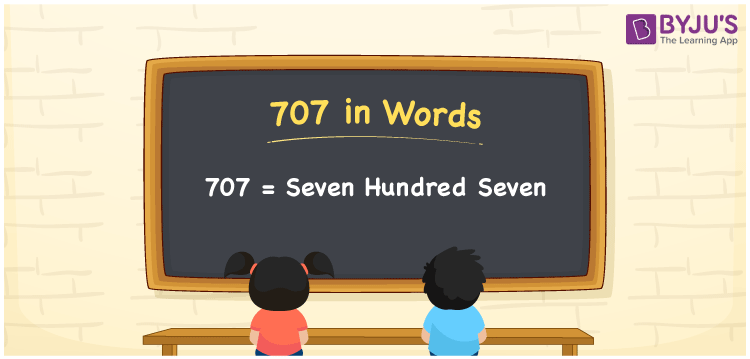# 707 in Words

707 in words is written as Seven Hundred Seven. The name of number 707 in English is “Seven Hundred Seven”. The word Seven Hundred Seven is used in many real-life situations. For example, there are Seven Hundred Seven people in a shopping mall. Thus, it is a counting number. Also, to represent the currency equal to 707, we can write it in words as Rupees Seven Hundred Seven or Seven Hundred Seven rupees. Therefore, it is necessary to learn the numbers in words, for the ease of understanding and expressing them.

 707 in words Seven Hundred Seven Seven Hundred Seven in Numbers 707

## 707 in English Words## How to Write 707 in Words?

If we know the place value of digits of 707, then we can easily express it in words. The place value is basically the position of a digit in a number. 707 is a three-digit number, therefore, we can specify the position of each digit of 707 in a place value chart. In the Indian numbering system, the order of place value of digits from right to left is given by:

 Hundreds Tens Ones 7 0 7

We can write it in expanded form as:

7 × Hundred + 0 × Ten + 7 × One

= 7 × 100 + 0 × 10 + 7 × 1

= 700 + 7

= 707

= Seven Hundred Seven

Therefore, 707 in words is written as eight hundred thirties.

707 is a natural number that precedes 708 and succeeds 706.

707 in words – Seven Hundred Seven

Is 707 an odd number? – Yes

Is 707 an even number? – No

Is 707 a perfect square number? – No

Is 707 a perfect cube number? – No

Is 707 a prime number? – Yes

Is 707 a composite number? – No

## Frequently Asked Questions on 707 in Words

Q1

### How to write 707 in words?

707 in English is written as “Seven Hundred Seven”.
Q2

### Is 707 an odd number or even number?

707 is an odd number because it is wholly not divisible by 2.
Q3

### Write Seven Hundred Seven in numbers.

Seven Hundred Seven in numbers is 707.